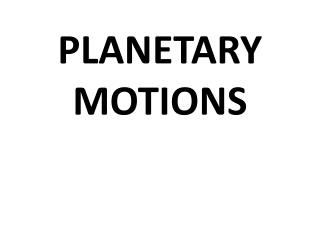DownloadDownload PresentationPLANETARY MOTIONS

# PLANETARY MOTIONS

Télécharger la présentation## PLANETARY MOTIONS

- - - - - - - - - - - - - - - - - - - - - - - - - - - E N D - - - - - - - - - - - - - - - - - - - - - - - - - - -
##### Presentation Transcript

1. PLANETARY MOTIONS

2. A few commonly confused terms: • 1. ROTATION is the motion of a planet SPINNING on its axis: • 1 complete rotation = 1 day • 2. REVOLUTION is the motion of a planet ORBITING its star (sun): • 1 complete revolution = 1 year

3. Planets are in motion around stars due to: • MOMENTUM: the force of motion; = mass x velocity • INERTIA: the tendency for objects to resist changes in motion: -Objects at rest remain at rest -Objects in motion remain in motion (at the same speed and direction - unless influenced by an outside force - THE LAW OF INERTIA)

4. Why do the planets move at all? • The solar system was “born” from a spinning nebula (gas/dust cloud) • so all of the objects in our solar system STILL have this MOMENTUM due to INERTIA • (objects in motion remain in motion - high speeds help too!)

5. THE ORBITS OF THE PLANETS • A planet’s ORBITAL PATH is the RESULTANT of the opposing forces of INERTIA AND GRAVITY • INERTIA: forces the planet to “try to” fly off into space in a straight line path - keeps planets from crashing into the sun • GRAVITY: pulls the planet towards the sun - prevents the planet from flying off into space in a straight line path

6. If the forces of INERTIA and GRAVITY are in balance, the planet will orbit the sun; if they are not balanced, it will spiral into the star, or fly out of the solar system

7. There is no such thing as a “curved path”

8. Planetary Orbits A planet’s “circular” or “elliptical” orbit actually consists of an infinite number of small, angled “turns” or changes of direction, which are the RESULTANT of the forces of gravity and intertia working against each other.

9. ORBITAL VELOCITY: VECTOR EXERCISES WORKSHEET

10. Diagram 1 The diagram to the left includes accurate GRAVITY VECTORS for the black and white planets as they orbit the gray star. 1. sketch INERTIA VECTORS of accurate lengths for each planet. 2. Explain why you sketched vectors of differing lengths for the planets:

11. The diagram to the left includes ONE INACCURATE VECTOR for ONE of the planets (both are correct for the 2nd planet) 1. Identify the incorrect planet and vector 2. . Explain what would happen to this planet if the vectors shown for gravity and inertia WERE CORRECT.

12. Diagram 3 The diagram to the left includes ONE INACCURATE VECTOR for ONE of the planets (both are correct for the 2nd planet) 1. Identify the incorrect planet and vector 2. . Explain what would happen to this planet if the vectors shown for gravity and inertia WERE CORRECT.

13. Orbits of the planets: strongest and weakest forces